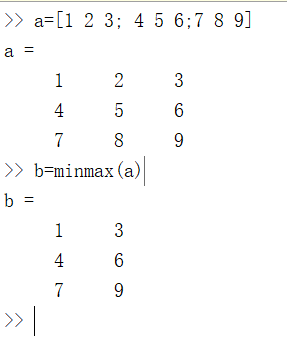• （1）C = max(A) 返回一个数组各不同维中的最大元素。 如果A是一个向量，max(A)返回A中的最大元素。 如果A是一个矩阵，max(A)将A的每一列作为一个向量，返回一个行向量,向量的第i个元素是矩阵A的第i列上的最大值。 ...

（1）C = max(A)
返回一个数组各不同维中的最大元素。
如果A是一个向量，max(A)返回A中的最大元素。
如果A是一个矩阵，max(A)将A的每一列作为一个向量，返回一个行向量,向量的第i个元素是矩阵A的第i列上的最大值。

（2）[Y,U]=max(A)：返回行向量Y和U，Y向量记录A的每列的最大值，U向量记录每列最大值的行号。

（3）max(A,[],dim)：返回A中有dim指定的维数范围中的最大值。
dim取1或2。

dim取1时，[Y,I]=max(M,[],1), 在第1维方向上取最大值，也就是每列最大值，结果存在Y里，I里存的是每列最大值的行位置。
该函数和max(A)完全相同；

dim取2时，[Y,I]=max(M,[],2), 在第2维方向上取最大值，也就是每行最大值，结果存在Y里，I里存的是每行最大值的列位置。
该函数返回一个列向量，其第i个元素是A矩阵的第i行上的最大值。

展开全文• func =Columns 1 through 80.0004 0.0004 0.0004 0.0003 0.0006 0.0004 0.0005 0.0004Columns 9 through 160.0009 0.0008 0.0007 0.0004 0.0018 0.0002 0.0004 0.0007Col...

func =

Columns 1 through 8

0.0004    0.0004    0.0004    0.0003    0.0006    0.0004    0.0005    0.0004

Columns 9 through 16

0.0009    0.0008    0.0007    0.0004    0.0018    0.0002    0.0004    0.0007

Columns 17 through 24

0.0007    0.0003    0.0017    0.0009    0.0003    0.0004    0.0005    0.0003

Columns 25 through 32

0.0005    0.0004    0.0003    0.0003    0.0002    0.0006    0.0005    0.0004

Columns 33 through 40

0.0007    0.0004    0.0003    0.0003    0.0002    0.0004    0.0004    0.0008

Columns 41 through 48

0.0005    0.0005    0.0005    0.0004    0.0003    0.0004    0.0006    0.0003

Columns 49 through 56

0.0009    0.0003    0.0006    0.0004    0.0008    0.0006    0.0005    0.0005

Columns 57 through 64

0.0002    0.0006    0.0004    0.0008    0.0005    0.0005    0.0007    0.0011

Columns 65 through 72

0.0005    0.0005    0.0006    0.0010    0.0004    0.0006    0.0003    0.0008

Columns 73 through 80

0.0004    0.0003    0.0003    0.0002    0.0008    0.0011    0.0005    0.0004

Columns 81 through 88

0.0006    0.0004    0.0003    0.0003    0.0003    0.0003    0.0005    0.0009

Columns 89 through 96

0.0004    0.0006    0.0007    0.0011    0.0004    0.0003    0.0005    0.0006

Columns 97 through 100

0.0008    0.0007    0.0004    0.0005

返回值是这个，帮我看看怎么回事，谢谢了

展开全文• Matlabmax函数在矩阵中求函数大小的实例如下:C = max(A)返回一个数组各不同维中的最大元素。如果A是一个向量，max(A)返回A中的最大元素。如果A是一个矩阵，max(A)将A的每一列作为一个向量，返回一行向量包含了每一...

Matlab中max函数在矩阵中求函数大小的实例如下:

C = max(A)

返回一个数组各不同维中的最大元素。

如果A是一个向量，max(A)返回A中的最大元素。

如果A是一个矩阵，max(A)将A的每一列作为一个向量，返回一行向量包含了每一列的最大元素。

如果A是多为数组，max(A) treats the values along the first non-singleton

dimension as vectors, returning the maximum value of each

vector.

C = max(A,B)

返回一个和A和B同大小的数组，其中的元素是从A或B中取出的最大元素。

C = max(A,[],dim)

返回A中有dim指定的维数范围中的最大值。

[C,I] = max(...)

找到A中那些最大值的索引位置，将他们放在向量I中返回。如果这里有多个相同最大值时，返回的将是第一个的索引。

-----------------------------------------------

max

Maximum elements of an array

Syntax

C = max(A)

C = max(A,B)

C = max(A,[],dim)

[C,I] = max(...)

Description

C = max(A) returns the largest elements along different dimensions

of an array. If A is a vector, max(A) returns the largest element

in A. If A is a matrix, max(A) treats the columns of A as vectors,

returning a row vector containing the maximum element from each

column. If A is a multidimensional array, max(A) treats the values

along the first non-singleton dimension as vectors, returning the

maximum value of each vector.

C = max(A,B) returns an array the same size as A and B with the

largest elements taken from A or B.

C = max(A,[],dim) returns the largest elements along the dimension

of A specified by scalar dim. For example, max(A,[],1) produces the

maximum values along the first dimension (the rows) of A.

[C,I] = max(...) finds the indices of the maximum values of A, and

returns them in output vector I. If there are several identical

maximum values, the index of the first one found is

returned.

Remarks

For complex input A, max returns the complex number with the

largest complex modulus (magnitude), computed with max(abs(A)), and

ignores the phase angle, angle(A). The max function ignores

NaNs.

isnan, mean, median, min, sort

展开全文• 选取每行中的最小值和最大值

选取每行中的最小值和最大值展开全文matlab
• 博客1--tensorflow的图像基本处理操作 话不多,具体内容在开源中国我的博客:https://my.oschina.net/u/3770644 代码: #!/usr/bin/env python# -*- coding:utf-8 -*-# ... npm太慢， 修改npm镜像 今天晚上想要将...
• max和min基础的用途是，求两数中最大的或最小的一个。 比如，max(a,b)。如果a>b,则返回值为a。min(a,b)则返回b。 求矩阵中每列的最大最小值 如果a是一个m行n列的矩阵。max(a)则求每列中的最大值，返回值为1行n...matlab max
• y1=max(double(y)); c=atan((y1)/x); x0=double(solve(((x0)-x)/(h+(y1)) == tan(2*c-a))); x1=double(solve(((x1)-x)/(h+(y1)) == tan(2*c+a))); if x0>20 light(n)=0; else if x1>(R/2) x1=20; for xin=x0:0.001:...
• matlab中，min和max可以用于求数值中的最小或最大值。 如： >> A=[1 2 8;4 7 6;2 6 7;5 6 1] A = 1 2 8 4 7 6 2 6 7 5 6 1 A为m×n矩阵。min(A)：表示的是每一列的最小值，为一个1*n的矩阵 >> ...最小值
• matlab中min max函数的用法 min Smallest component. For vectors, min(X) is the smallest element in X. For matrices, min(X) is a row vector containing the minimum element from each column. For N-D arrays...tensorflow 人工智能 python
• model3d/@model3d/model3d/@model3d/center_of_mass.mmodel3d/@model3d/greyscale.mmodel3d/@model3d/layer_names.mmodel3d/@model3d/magnify.mmodel3d/@model3d/minus.mmodel3d/@model3d/model3d.dllmodel3d/@model...
• 好吧,这有点涉及,但你可以获得所有最大值的索引,然后使用randi和accumarray随机选择一个：%# (1) Find the maxima%# if you are interested in the global maximum%# that may occur ...maxIdx] = max(Defender....matlab
•matlab
• a = max(A); 如果A是一个向量，max(A)返回A中的最大元素。 如果A是一个矩阵，max(A)将A的每一列作为一个向量，返回一行向量包含了每一列的最大元素。 坑 之前一直以为不管是向量还是矩阵都是返回A中的最大元素；...matlab
• Max pooling layer

2021-04-26 16:29:32
maxPooling2dLayerDescriptionA max pooling layer performs downsampling by dividing the inputinto rectangular pooling regions, and computing the maximum of each region.CreationDescriptionlayer = maxPool...
• matlab中的最大值和最小值

千次阅读 2021-04-18 09:03:00
matlab中的的最大值和最小值MATLAB提供的求数据序列的最大值和最小值的函数分别为max和min，两个函数的调用格式和操作过程类似。1．求向量的最大值和最小值求一个向量X的最大值的函数有两种调用格式，分别是：(1) y=...
• 你在交叉训练的时候错误最多能有多少个 好想默认是6个
• Matlabmax函数在矩阵中求函数大小的实例如下:C = max(A)返回一个数组各不同维中的最大元素。如果A是一个向量，max(A)返回A中的最大元素。如果A是一个矩阵，max(A)将A的每一列作为一个向量，返回一行向量包含了每一...
• Matlabmax函數在矩陣中求函數大小的實例如下:C = max(A)返回一個數組各不同維中的最大元素。如果A是一個向量，max(A)返回A中的最大元素。如果A是一個矩陣，max(A)將A的每一列作為一個向量，返回一行向量包含了每一...
• R shi 3*3 *3 R[;] 变成一行 max默认只会是max(A,1) sort（有个参数是降序）’descend
• [编辑:整个问题有一个非常简单的解决方案:矩阵使用单一数据类型而不是默认的双精度类型]我刚刚注意到Matlab中的一个(我认为)异常行为,想知道是什么导致了它。我有一个10000x500矩阵m,其值范围为min(min(M)) = -226....
• argmin(matlab中argmin函数)

千次阅读 2021-01-27 07:51:31
matlab有概率统计工具包，但是. 经常碰到诸如f(x)=arg min{....}，是不是就是令f(x)等于min{....} ??? argmax表示寻找具有最大评分的参量 函数y=f(x),x0= argmax(f(x)） 的意思就是参数x0使得f(x0)为f(x)的最大值；...
• 我使用MATLAB的 「fminimax」 解決以下問題：在MATLAB中搜索max-minki=G(i,:);ki(i)=0;fs(i)=-((G(i,i)*pt(i)+sum(ki.*pt)+C1)-(C2*(sum(ki.*pt)+C1)));G：是一個系統矩陣。 pt：是優化變量。當使用實際系統矩陣時...
• MATLAB中BP神经网络的重要函数和基本功能 ?newff() 功能 建立一个前向BP网络 格式 net = newff(PR,[S1 S2...SN1],{TF1 TF2...TFN1},BTF,BLF,PF) ......( 湖北民族学院 理学院 湖北 恩施 445000) 摘 : 为满足工程应用...
• 简单,易懂Matlab中的size函数size(A)函数是用来求矩阵的大小。比如说一个A是一个3×4的二维矩阵： 1、size(A) %直接显示出A大小 ans=3 42、s=size(A)%返回一个行向量s，s的第一个元素是矩阵的行数，第二个元素是矩阵...
• 本篇主要通过几个例子来介绍一些常用的MATLAB预定义函数,MATLAB中的数学函数在很大程度上保留了原有的数学格式，所以在使用和学习起来是非常方便的，相信大家在下面的例子中会体会到这一点。基本数学函数abs(x)计算x...
• minmax(P_train)得到矩阵P_train的最小和最大值(找到每行的最小和最大,有多少行就有多少对最小和最大)；[17,3]意思是在隐层建立17个神经元,输出层建立一个神经元；'tansig','logsig'},'traingdm'是神经网络的一些...
• clc;clear;close all;PATH = 'C:\Users\UserName\Desktop\Img_20200509\';fileFolder=fullfile(PATH);dirOutput=dir(fullfile(fileFolder,'*.jpg'));[size_1, size_2] = size(dirOutput);e = actxserver('Excel.Appl...
• 以下以min()函数为例，max()函数同理 求矩阵A的最小值的函数有3种调用格式，分别是： (1) min(A)：返回一个行向量，向量的第i个元素是矩阵A的第i列上的最小值。 (2) [X,Y]=min(A)：返回行向量X和Y，X向量记录A的每列...matlab
• 该楼层疑似违规已被系统折叠隐藏此楼查看此楼首先，我先描述一下我的问题，我得到了一个三维数组，数字代表了三维空间中的势能，我想把等势面画出来，也就是把势能相同的点连接起来形成面，问下有没有大牛会的，之前......

matlab里的maxmatlab 订阅